# Computations

Theorem 3 can be directly translated into an algorithm that computes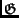-families. The algorithm simply computes the coset leaders of-codes in the family, from which the remaining parameters may be easily deduced. This computation scheme has the advantage of being exponential in the co-dimension (i.e., the dimension of the dual code) rather than the dimension or the length. Thus, this algorithm is efficient for high-rate codes, and it is presented in Algorithm 1.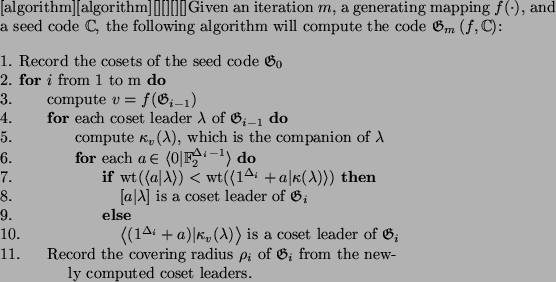In Algorithm 1 we reuse our earlier notation that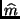. Note that we assume that the coset leaders of the seed code are known or trivially derivable, as is often the case. The correctness of the algorithm follows immediately from Theorem 3. Moreover, we can also compute the running time and space of this algorithm in terms of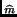nm - m, the co-dimension of the m-th-code,

Lemma 4   Using an oracle for computing the generating mapping, Algorithm 1 runs in space O(2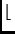) and time O(nmm2).

An oracle is simply a black box that computes a given function in constant time. In this case, we assume the existence of an oracle for computing the generating mapping because the complexity of such a computation can vary greatly among mappings. The proof of Lemma 4 follows from a straightforward analysis of the pseudo-code for Algorithm 1.

For the specific case of distance 4 lexicodes, this algorithm is particularly fast, since Brualdi and Pless [3, Thm.3.5] show that, under these circumstances:

 m = nm - 2 -log2(nm - 1)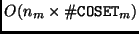(13)

so that Lemma 4 reduces to time O(nmm log(nm)) and space O(nm). This is not surprising given that the distance 4 lexicodes are simply shortenings of the extended Hamming codes .

The analysis of Algorithm 1 assumes an oracle computation of the generating mapping. For the case of lexicodes and trellis-oriented lexicodes, however, Method 1 provides an efficient means of computing this mapping in time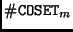and O(nm) space, whererepresents the number of cosets in the m-th corresponding-code. As in the case of lexicodes and trellis-oriented codes, the overhead of computingis usually eclipsed by the running time of the remainder of the algorithm.

Corollary 3   Algorithm 1 requires time O(nmm2) and space O(2) to compute lexicodes and trellis-oriented lexicodes.

The complexity of Algorithm 1 is thus bounded by the co-dimension of the code and by the difficulty of computing the generating mapping. Under practical conditions, we were able to compute lexicodes well beyond length 44 initially reported in .

In addition, we were able to construct trellis-oriented codes with code parameters rivaling those of lexicodes, but with much better trellis state complexities. As an example, we generated a trellis-oriented-code with code parameters similar to lexicodes, but with better state complexity than the corresponding BCH codes heuristically minimized in . These result were predicted by  and are demonstrated in Table 4.

Table 4: A comparison of the trellis state complexities of the (31, 16, 7) trellis-oriented lexicode (TRE) and a (31, 16, 7) extended BCH code (BCH) heuristically minimized in  .
 TRE: 0 : 1 : 2 : 3 : 4 : 5 : 6 : 6 : 7 : 8 : 9 : 8 : 9 : 8 : 7 : 6 : 7 : 6 : 6 : 6 : 5 : 5 : 4 : 3 : 4 : 4 : 4 : 3 : 3 : 2 : 1 : 0 BCH: 0 : 1 : 2 : 3 : 4 : 5 : 6 : 6 : 7 : 8 : 9 : 8 : 9 : 8 : 7 : 6 : 7 : 8 : 9 : 8 : 9 : 8 : 7 : 6 : 7 : 6 : 5 : 4 : 3 : 2 : 1 : 0

Finally, the trellis state bounded codes that we have computed show improvements, for various code parameters, over the state-bounded codes computed in  using different techniques.